# 06-1350/Syzygies in Asymptote

For a condensed version of this page, check 06-1350/Syzygies in Asymptote in Brief.

## Contents

### Disclaimer

These instructions (and the program they describe) are a work in progress and should be considered highly unreliable.

### Installation

To use the syzygy script, you need to have a working version of Asymptote. Asymptote is installed on coxeter. To install on another computer, see Asymptote. Instructions for installing the program on several OSes is given in the documentation at the Asymptote website. The documentation also gives helpful instructions on how to run a script in Asymptote to produce a picture. You should also have (or install) a variant of TeX on your system, such as MiKTeX, so that Asymptote can typeset labels. The syzygy package is now part of the standard Asymptote distribution.

### Braids

Once installed, we can draw a braid in Asymptote:

import syzygy;  // Accesses the syzygy module.
Braid b;        // Start a new braid.
b.n=3;          // The braid has three strands.
// The strands are numbered left to right starting at 0.
// the leftmost strand.
b.add(phi,0);   // Add a trivalent vertex that merges strands 0 and 1.
// Strand 2 is now renumbered as strand 1.
b.draw();       // Draw the resulting braid.


When saved into an asy file, say mybraid.asy and run with Asymptote, the result is a picture:

### Relations

#### Drawing

To define a relation, we first define two braids, and then stick them into a Relation structure. The below script generates an R3 relation.

import syzygy;      // Access the syzygy module.
Braid l;            // Define the left hand side of the relation.
Braid r;            // Define the right hand side of the relation.

Relation r3;        // Define a relation.
r3.lsym="\rho_3";   // Give the relation a name for when it is written in functional form.
r3.codename="rho3"; // Give the relation a name to be used by Mathematica.
r3.lhs=l;  r3.rhs=r;
r3.draw();


When saved into an asy file and run, this draws the two sides of the relation. If TeX is installed, Asymptote will also put a lovely equals sign, typeset by TeX, between the two figures.

#### Outputting Equations

We can also get useful equations out of the relation. The method r3.toFormula() will produce a string that is the formula for the relation.

(1230)^\star B^+ (1213)^\star B^+ (1023)^\star B^+ =
(1123)^\star B^+ (1203)^\star B^+ (1231)^\star B^+


This string can be written out to the standard output by write(r3.toFormula()). It can be written to a file by file f=output("filename.txt"); write(f, r3.toFormula()). The string is formatted so it can be put into TeX or a wiki page using math mode: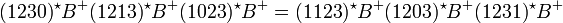$(1230)^\star B^+ (1213)^\star B^+ (1023)^\star B^+ = (1123)^\star B^+ (1203)^\star B^+ (1231)^\star B^+$

The method r3.toLinear() produces the formula in linear form: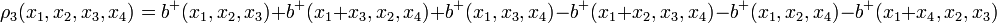$\rho_3(x_1,x_2,x_3,x_4) = b^+(x_1,x_2,x_3) + b^+(x_1+x_3,x_2,x_4) + b^+(x_1,x_3,x_4) - b^+(x_1+x_2,x_3,x_4) - b^+(x_1,x_2,x_4) - b^+(x_1+x_4,x_2,x_3)$

and r3.toCode() produces a version of the relation that can be used in Mathematica:

rho3[x1_, x2_, x3_, x4_] :> bp[x1, x2, x3] + bp[x1 + x3, x2, x4] + bp[x1, x3, x4]
- bp[x1 + x2, x3, x4] - bp[x1, x2, x4] - bp[x1 + x4, x2, x3]


A few relations, such as r3, are already defined in syzygy.asy but more should be added.

#### Applying

Now that we have relations, we can apply them to bigger braids. Let's start with the braid in the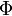$\Phi$ around B syzygy:

import syzygy;
Braid b;
b.n=4;


After skipping the lowest knot, we can apply R3 to the next three knots:

Braid bb=apply(r3, b, 1, 0);


here apply(r, b, k, n) means we are applying the relation r to the braid b at the place in the braid found by counting k components up from the bottom component and n strands in from the leftmost strand. apply does not modify the original braid, but returns the result of applying the relation (stored here as bb):

This went from the left hand side of the relation to the right hand side. To apply a relation in reverse, simply prefix it by a minus sign. For example apply(-r3, bb, 1, 0) will yield a braid equivalent to our original. When applying a relation, the script first checks that the one side of the relation matches that portion of the braid, and will give a (somewhat cryptic) error if the relation cannot be applied.

In our braids, the components are placed from bottom to top in a fixed order. Sometimes when building syzygies, it is neccessary to swap the order that these components occur. This is done by the swap method. For instance, starting from b, we can swap the two bottom crossings:

Braid swapped=b.swap(0,1);


Remember that components are ordered from bottom to top, starting at 0. Again, the script checks to make sure the swap is valid (ie. changing the order of the two components, doesn't actually change the knot) and will issue an error if it isn't.

### Syzygies

One could manually apply relations and swaps, and make a whole bunch of braids, but it would be annoying to keep track of them all. Thankfully, the Syzygy structure does that for us. For example, here is the complete code for the$\Phi$ around B syzygy:

import syzygy;

// Phi around B
Braid initial;
initial.n=4;

Syzygy pb;
pb.lsym="\Phi B";
pb.codename="PhiAroundB";
pb.initial=initial;
pb.apply(r3,1,0);
pb.apply(r4a,3,1);
pb.swap(2,3);
pb.apply(r4b,0,1);
pb.apply(-r3,1,0);
pb.apply(-r4a,0,0);
pb.swap(2,3);
pb.apply(-r4b,3,0);
pb.apply(r3,1,1);

pb.draw();


and the result

Again, like relations, we can use pb.toLinear()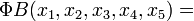$\Phi B(x_1,x_2,x_3,x_4,x_5) =$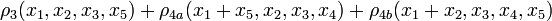$\rho_3(x_1,x_2,x_3,x_5) + \rho_{4a}(x_1+x_5,x_2,x_3,x_4) + \rho_{4b}(x_1+x_2,x_3,x_4,x_5)$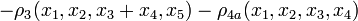$- \rho_3(x_1,x_2,x_3+x_4,x_5) - \rho_{4a}(x_1,x_2,x_3,x_4)$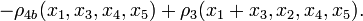$- \rho_{4b}(x_1,x_3,x_4,x_5) + \rho_3(x_1+x_3,x_2,x_4,x_5).$

and pb.toCode()

PhiAroundB[x1_, x2_, x3_, x4_, x5_] :> rho3[x1, x2, x3, x5] + rho4a[x1 + x5, x2, x3, x4]
+ rho4b[x1 + x2, x3, x4, x5] - rho3[x1, x2, x3 + x4, x5] - rho4a[x1, x2, x3, x4]
- rho4b[x1, x3, x4, x5] + rho3[x1 + x3, x2, x4, x5]


to give the formulas for the syzygies.

The Syzygy structure assumes that after the last application of a relation, the braid is in the same form as the start, so it won't draw the last braid. This is annoying when building a syzygy, so it can be turned off by pb.cyclic=false; If you set bp.showall=true; the syzygy will draw all changes to the braid, including swaps. Finally, setting bp.number=true; will print numbers on the diagrams so you can follow them around.

### Conclusion

Example syzygyies and the latest version of the script can be found in the syzygy directory on the course Subversion repository . Please contact me if you have any questions or suggestions. Good luck and happy syzyging!